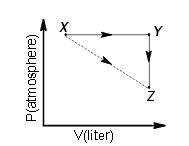Courses

# Notes | EduRev

## JEE : Notes | EduRev

The document Notes | EduRev is a part of the JEE Course NEET Revision Notes.
All you need of JEE at this link: JEE

Question 1: A piston filled with 0.04 mol of an ideal gas expands reversibly from 50.0 mL to 375 mL at a constant temperature of 37.00 C. As it does so, it absorbs 208J of heat. The values of q and w for the process will be:
(R = 8.314 J/mol K) (ln 7.5 = 2.01)    (IIT-JEE 2013)
(a) q = – 208 J, w = – 208 J
(b) q = – 208 J, w = + 208 J
(c) q = + 208 J, w = + 208 J
(d) q = + 208 J, w =– 208 J
Solution:
For isothermal process, ? U = 0
Process is isothermal reversible expansion, hence dE =0
U = 0.
So, Using 1st law of thermodynamics
q = -W
or
w = -q = -208 J

Question 2: For an ideal gas, consider only P-V work in going from an initial state X to the final state Z. The final state Z can be reached by either of the two paths shown in the figure. Which of the following choice(s) is(are) correct
[Take ?S as change in entropy and was work done]    (IIT-JEE 2012)(a) ΔS x→z = ΔS x→y + ΔS y→z
(b) w x→z = w x→y + w y→z
(c) w x→y→z = w x→y
(d) ΔS x→y→z = ΔS x→y
A & C
Solution:
Entropy  being a state function shows additive property.
Hence,
ΔS x→z = ΔS x→y + ΔS y→z
For the process Y →Z pressure is constant.
W = -pΔV
At constant pressure
W =0
Hence, w x→y→z = w x→y

Question 3: The standard enthalpies of formation of CO2(g), H2O(l) and glucose(s) at 25 0C are–400 kJ/mol, –300 kJ/mol and –1300 kJ/mol, respectively. The standard enthalpy of combustion per gram of glucose at 250C is    (IIT JEE 2013)
(a) +2900kJ
(b) -2900 kJ
(c) +16.11 kJ
(d) -16.11 kJ
Solution:
Formation of CO2:
C(graphite) + O2 (g)→ CO2 (g)    ΔH1= -400 kJ ………….(1)
Formation of H2O:
H2(g) + O2 (g) → H2O (l)    ΔH2= -300 kJ…………..(2)
Formation of C6H12O6:
6C( graphite) + 3O2 (g) + 6H2( g)  → C6H12O6 (s)    ΔH3= -1300 kJ ………(3)
6×Equation (1) + 6×Equation (2) - Equation (3)
We get,
C6H12O6 (s)    + 6O2 (g) → 6CO2 (g) + 6H2O (l)    ……….(4)
ΔH4= 6×(-400) + 6×(-300)- (-1300) = 2900 kJ/mol
Equation number 4 represents the equation for combustion of 1 mole of glucose so, the
enthalpy change for the reaction i.e. ΔH4 is the enthalpy of combustion of glucose.
Enthalpy change for combustion of 1 mole i.e. 180 gm glucose = 2900 kJ
So, enthalpy change for combustion of 1 gm glucose =  2900/180 = 16.11 kJ.
Hence, the correct option is c.

Offer running on EduRev: Apply code STAYHOME200 to get INR 200 off on our premium plan EduRev Infinity!

291 docs

,

,

,

,

,

,

,

,

,

,

,

,

,

,

,

,

,

,

,

,

,

;